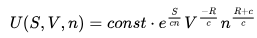Internal Energy - ThermodynamicsIn thermodynamics, the internal energy is the total energy contained in a thermodynamic system.

The internal energy is the energy that is needed to create the system. According to this definition, the energy to displace the environment of the system, any energy related to external force fields (potential energy, gravitational energy, etc.) or any energy associated with the movement (for example, kinetic energy) is excluded from the internal energy. .

The internal energy of a system can be modified by exercising a work on it or by heating it (providing thermal energy). If we look at the first law of thermodynamics we see that this postulates that the increase in internal energy is equal to the total heat added plus the work done by the environment. If the system is isolated, its internal energy remains constant.

The internal energy is a function of the state of the system, since its value depends only on the current state of the system and not on the chosen path to reach it. It is an extensive property.

An example to understand what is internal energy we find in nuclear weapons. The energy released by nuclear weapons is a very small part of your internal energy. However, they emit tremendous energy and have great devastation.

Internal kinetic energy and internal potential energy

The internal energy is the sum of two different types of energy: the internal kinetic energy and the internal potential energy.

The internal kinetic energy is the sum of the kinetic energies of each element of a system with respect to its center of masses. The internal kinetic energy is caused by the movement of the particles of the system (translations, rotations and vibrations).

The potential internal energy is the potential energy associated with each of the interactions of these elements. The internal potential energy is associated with the static constituents of matter, the electrostatic energy of the atoms within the molecules or crystals, and the static energy of the chemical bonds.

Internal energy of the ideal gas

Thermodynamics often uses the ideal gas concept. This concept is an approximation of the real systems used for didactic purposes.

The ideal gas is a gas of particles considered as point objects that interact only by elastic collisions and fill a volume in such a way that its average free travel between collisions is much larger than its diameter. Such systems are approximated by monatomic gases, helium and other noble gases. Here the kinetic energy consists only in the energy of translation of the individual atoms. The monatomic particles do not rotate or vibrate, and they are not excited electronically at higher energies, except at very high temperatures.

Therefore, changes of internal energy in an ideal gas can only be described by changes in its kinetic energy. Kinetic energy is simply the internal energy of the perfect gas and depends completely on its pressure, volume and thermodynamic temperature.

The internal energy of an ideal gas is proportional to its mass (number of moles) n and its temperature T

U = c · n · T

where c is the heat capacity (at constant volume) of the gas. The internal energy can be written in terms of the three extensive properties S, V, n (entropy, volume, mass) as followswhere const is an arbitrary positive constant and where R is the universal gas constant.

Change in internal energy

Direct measurement of internal energy is not possible, but the change in internal energy can be measured. This change can be found by measuring thermal energy, light energy or similar energies transmitted from the limits of internal energy to the environment, or by calculating the amount of work done by the system or the work done in the system. The change in internal energy is expressed by ΔU.

• System of an absorption energy occurs when an increase of domestic energy. The internal energy can be increased by doing work in the system.
• The internal energy decreases when the system emits an energy or works.

Changes in the thermal energy of a thermodynamic system

The system does not really have thermal energy; The internal energy is transferred to the medium in the form of thermal energy. In cases where a change in the internal energy of the system occurs due to the transfer of thermal energy, a very small portion of the internal energy changes.

When a thermodynamic system transfers thermal energy to another system, in addition to varying the internal energy the two systems can experience alterations of their other state variables (pressure, volume, temperature, enthalpy, entropy ...)

Units of measurement of internal energy

The unit of measurement of internal energy, according to the International System, is July (J).

Sometimes we talk about the specific internal energy. The specific internal energy is the internal energy per unit mass; Your unit is J / kg. You can also define the intensive property molar internal energy, which expresses the internal energy in relation to the amount of substance; its units are J / mol.

valoración: 2.7 - votos 3

Last review: April 4, 2017

Back